﻿ Intrinsic Viscosity Determination of High Molecular Weight Biopolymers by Different Plot Methods. Chia Gum CasePublications are Open
Access in this journal
Article Versions
Export Article
• Normal Style
• MLA Style
• APA Style
• Chicago Style
Review Article
Open Access Peer-reviewed

### Intrinsic Viscosity Determination of High Molecular Weight Biopolymers by Different Plot Methods. Chia Gum Case

Martin A. Masuelli
Journal of Polymer and Biopolymer Physics Chemistry. 2018, 6(1), 13-25. DOI: 10.12691/jpbpc-6-1-2
Received August 17, 2018; Revised September 28, 2018; Accepted October 08, 2018

### Abstract

The chia (Salvia hispanica) generates an abundant and viscous mucilage, this is purified with periods of heating-cooling and finally precipitated with ethanol, obtaining chia gum, CG. In this work the intrinsic viscosity is determined by different methods being Huggins taken as standard. The different methods are compared and evaluated with their respective percentage relative errors. By means of intrinsic viscosity is determined the molecular weight with a value of 3846000g/mol. This polysaccharide acquires a rod-like conformation with an a value, Mark-Houwink parameter, of 0.803 according to Int. J. Biological Macromol. 81 (2015) 991-999. This macromolecule is very promising and has a potential in several industrial applications such as film forming, gel, thickener, and coemulsifier.

### 1. Introduction

Chia (Salvia hispanica) is an herbaceous plant of the family Lamiaceae, is of Mesoamerican origin; it is one of the plant species with the highest concentration of omega 3 (alpha-linolenic fatty acid). It is therefore cultivated to take advantage of its seeds, which are used as ground food 1. The seeds soaked in water release the mucilage, producing a gelatinous liquid; in Mexico it is flavored with vegetable juices or essences and it is consumed as a refreshing drink. The seeds can also be dried and ground to prepare a fine flour with an intense flavor, called pinole, which is consumed mainly as a sweet. Tender shoots are eaten as raw or cooked vegetables and can be used in salads. The nutritional composition of chia seed is: 20% protein, 40% dietary fiber (5% soluble fiber of very high molecular weight) and 34% oil; over 64% of the oil are omega 3 and fatty acids. It does not contain gluten, so it is suitable for celiacs. Non toxic components are known in it 2.

Lin et al 3, reported a tentative structural linear unit of chia seed gum, this is a tetrasaccharide with 4-O-methyl-a-D-glucoronopyranosyl residues occurring as branches at 0-2 of some b-D-xylopyranosyl residues in the main chain consisting of (1->4)-b-D-xylopyranosyl-(1->4)-a-D-glucopyranosyl-(1->4)-b-D-xylopyranosyl units. The molecular weight varies from 0.8 to 2.0 x 10 6 daltons.

Campos et al. 4, studied extraction of mucilage of chia seed varied temperature (30-80 C), extraction time (2-4 h) and water: seed ratio (10:1-30:1) on the yield.

Salgado-Cruz et al. 5, released microstructural characterization of chia seed mucilage. Results showed that the mucilage is excreted from the polygonal cells of the epidermis coat, being composed mainly by carbohydrates fibres (18-45 nm width).

Capitani et al. 6, evaluated the viscoelasticity and flow behavior of aqueous dispersions with different concentrations of chia mucilage (Salvia hispanica L.) from Argentina seeds. The mucilage obtained by two methods: (I) soaking-freezing-freeze drying-sieving, and (II) soaking-filtration-concentration-freezing-freeze drying. The effect of mucilage concentration, temperature, pH, ionic strength and presence of sucrose on the rheological properties of the aqueous dispersions with the addition of NaCl or CaCl2 is also evaluated.

Timilsena et al. 7, 8, extracted chia seed gum, and its rheological and microstructural properties in aqueous solutions are studied. The intrinsic viscosity is high (∼1600 cm3/g) Controlled acid hydrolysis of purified CSP yielded various low molecular fractions with fairly uniform polydispersity giving a Mark-Houwink relationship of intrinsic viscosity equaling to 0.0152 (molecular weight) 0.803 (cm3/g).

Capitani et al. 9, characterized the physicochemical and functional properties of meals (M) and fibrous fractions (FRF) of chia seeds (Salvia hispanica L.), and to compare the effect of oil extraction methods (pressing -p- and solvent extraction -s-) and sieving process on these properties.

Muñoz et al. 10, researched the effect of temperature (4-80 C), pH (4-8) and seed:water ratio (1:20 and 1:40) on extraction of the mucilage of chia seeds and the effect of temperature (20-80 C), pH (3-9) and ionic strength (0-1%) on hydration of the extracted mucilage. The mucilage is localized in cellular structures in the first three layers of the seed coat and upon full hydration filaments (mucilage fibers) became apparent and conformed to a transparent ‘‘capsule’’ attached to the seed. During extraction, temperature and seed:water ratio were found to have a significant effect on yield. Hydration of the extracted mucilage is significantly increased at high pH values, and was higher when salt concentration decreased, being maximal when the temperature reached values close to 80 C.

Segura-Campos et al. 11, extracted Chia Gum from its dietary fiber fractions for use as an additive to control viscosity, stability, texture, and consistency in food systems. They reported that gums dispersion exhibited a non-Newtonian fluid behavior, specifically shear thinning or pseudoplastic type.

Goh et al. 12, obtained hydrated chia seeds using water and isolated by ethanol precipitation. They reported that chia gum from freeze-dried consisted of ∼95% non-starch polysaccharides (35% w/w neutral soluble fraction and 65% w/w negatively charged insoluble fraction). The soluble polysaccharide fraction has molar mass, root-mean square radius and intrinsic viscosity of ∼5 × 105 g/mol, 39 nm and 719 cm3/g, respectively. The viscosity of the dispersion is fairly resistant to variations in temperatures (20-80°C), pH (4-12), ionic strengths (0.01-0.5 M NaCl) and cation types (MgCl2, CaCl2, NaCl and KCl). The swollen microgel particles dispersed in soluble polysaccharide continuous phase provided complex and potentially useful rheological properties in food systems.

Coorey et al. 13, studied the characteristics of chia gel and compared to guar gum and gelatin which are commonly used in the food industry. The extracted chia gels from seeds and flour are analyzed for moisture, ash, protein, crude fiber, oil, and fatty acid profile. Water-holding capacity, oil-holding capacity, viscosity, emulsion activity, and freeze-thaw stability of the extracted chia seed gel are similar to guar gum, and gelatin. Chia gel is a polysaccharide based gel mainly consists of crude fiber (58%) and carbohydrate (34%). Extracted chia seed gel has a great potential in food formulations as thickening agent, emulsifying agent, and as a stabilizer.

Dick et al. 14, investigated the physicochemical and mechanical properties of a novel edible film based on chia mucilage (CM) hydrocolloid. CM (1% w/v) films were prepared by incorporation of three concentrations of glycerol (25%, 50%, and 75% w/w, based on CM weight). As glycerol concentration increased, water vapor permeability (WVP), elongation at break, and water solubility of CM films increased while their tensile strength, and Young’s modulus decreased significantly. CM films exhibited excellent absorption of ultraviolet light, and good thermal stability. This study demonstrated that the chia mucilage hydrocolloid has important properties and potential as an edible film, or coating.

In this work we will study the mucilage obtained from the chia, which we purified for obtained of chia gum (CG). At CG we will perform physicochemical studies in aqueous solution using viscosity and density determinations. In this work the intrinsic viscosity is determined by different methods being Huggins taken as standard. The different methods are compared and evaluated with their respective percentage relative errors. Also, we will obtain Mark-Houwink parameters from intrinsic viscosity measurements and with which we will determine the molecular weight and the hydrodynamic parameters of CG.

1.1. Intrinsic Viscosity and Hydrodynamic Parameters

The viscosity of a capillary viscometer can be calculated from the following equation: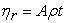(1)

It should also be remembered that: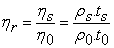(2)

where the subindex “s” indicates “solution” and “0” indicates “solvent” viscosity.

IUPAC recommends the term “increment of relative viscosity (ηi)”, instead of “specific viscosity”, because it has no attributions of specific quantity, meaning: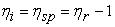(3)

when high concentrations are used it is better to start with the first term of the Huggins equation ηsp/c = ηred.

In Huggins’ method 15, intrinsic viscosity [η] is defined as the ratio of the increase in relative viscosity (ηsp) to concentration (c in g/cm3) when the latter tends towards zero.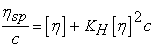(4)

where KH is Huggins constant.

The Kraemer 16 propose the following equation: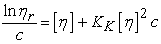(5)

where KK is Huggins constant.

The Martin 17 propose the following equation: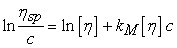(6)

where KM is Martin’s constant.

Fuoss 18 propose the following equation: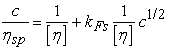(7)

where KFs is Fuoss constant.

Fedors 20 propose the following equation: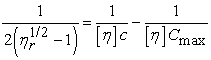(8)

where Cmax is polymer parameter concentration.

Heller 21 propose the following equation: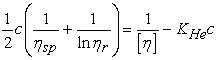(9)

where KHe is Heller’s constant.

Lyons & Tobolsky 22, 23 propose the following equation: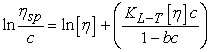(10)

where KL-T and b are constant.

Baker 24, 25 propose the following equation: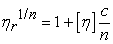(11)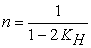(12)

with 0.25<KH<1, and KH = 0.9204.

Tager 26 propose the following equation: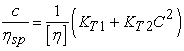(13)

where KT1 and KT2 are Tager’s constant.

Budtov 27 propose the following equation: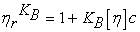(14)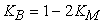(15)

Where KM is Martin's constant. This method is not applicable to intrinsic viscosity and molecular weights as high and KM>0.5.

Solomon & Gotesman 28, 29, 30 propose the following equation: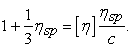(16)

Arrhenius-Rother-Hoffmann 31, 32, 33, 34 propose the following equation: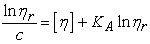(17)

where KA is constant.

Kreisa 35 propose the following equation: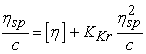(18)

where KKr is Kreisa’s constant.

Staudinger & Heuer 35 propose the following equation: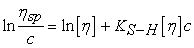(19)

where KS-H is a constant.

Schramek 37 propose the following equation: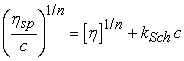(20)

where KSch is Schramek’s constant.

Maron & Reznik 38 propose the following equation: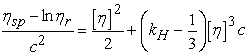(21)

Maron-Reznik Modified equation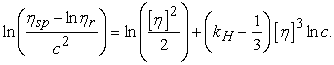(22)

In this work two alternatives are proposed that serve to calculate the intrinsic viscosity and are models that take into account the average between two values obtained by different methods that we will detail next.

The first is a plot method where the figures of the equations are made 23 and 24. Finally, from the ordinate to the origin, the intrinsic viscosity is obtained and the average is obtained from them.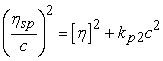(23)

The relative percentage error (RE%) with respect to Huggins of this equation is by excess.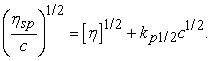(24)

The percentage relative error (RE%) with respect to Huggins of this equation is by default.

Therefore, an average of both plot solutions gives a value of less than 5% regarding the Huggins method.

In the second case, an empirical equation is proposed, which is a combination of both, from the realization of the figure and from the ordinate to the origin, the intrinsic viscosity is obtained. This work proposes the following empiric equation: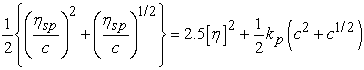(25)

where kp2, kp1/2 and kp are constants which contain the intrinsic viscosity function.

The Mark-Houwink 39, 40 equation (26) describes the relationship between intrinsic viscosity and molecular weight. Since molecular weight is related to the size of the polymer chain 41. The calculation of Mark-Houwink (M-H) parameters is carried out by the plot representation of the following equation: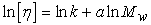(26)

where k and a are M-H constants, depending upon the type of polymer, the solvent, and the temperature of viscometric determinations. The exponent a is a function of polymer geometry and varies from 0.5 to 2.0. These constants can be determined experimentally by measuring the intrinsic viscosity of several polymer samples for which the molecular weight has been determined by an independent method (e.g., osmotic pressure or light scattering) 42.

Using the polymer standards, a plot of ln [η] versus ln Mw usually gives a straight line. The slope of this line is the value of a and its intercept is equal to ln k. The M-H exponent bears the signature of a polymer chain's three-dimensional conformation in the solvent environment: a values from 0.0−0.5 reflect a rigid sphere in an ideal solvent; those from 0.5−0.8 a random coil in a good solvent; and from 0.8−2.0 a rigid or rod like configuration (stiff chain). The polymer molecule assumes a tighter configuration, and the solution has a lower intrinsic viscosity. The M-H “a” constant is close to 0.5 in “poor” solvents, whereas for a rigid or rod-like polymer molecule that is greatly extended in solution the “a” constant approaches a value of 2.0 43, 44.

The hydrodynamic radius (RH) is given by the Einstein relation 45,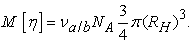(27)

A common practice to express the intrinsic viscosity and specific volume 46, as: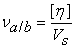(28)

ν(a/b) is called Einstein viscosity increment, and Vs is specific volume (cm3/g) 47, 48.

### 2. Experimental

2.1. Mucilage Chia Gum (MCG)

The mucilage was collected with a syringe from the seeds of chia, Salvia hispanica. To dissolve this seeds, heating-cooling periods were carried out in agitation during 8hs at 90°C. Once dissolved, it was pressure-filtration and precipitated with ethanol several times. Finally, it was dried at 60°C for 24hs, and redissolved in distilled water at a concentration of 0.1% wt.

• Figure 1. Salvia hispanica flower, seed and mucilage
2.2. Viscosity and Density

Measurements were taken from fresh MCG in aqueous solutions of 0.001-0.1 % wt. Solutions and dissolutions were prepared with deionised water. The different temperatures were maintained using a HAAKE C thermostatic bath (±0.1°C). Determinations were done using an Ubbelohde “suspended level” viscometer (IVA 1), with a water draining time of 35.97s. The density of each solution was measured using an Anton Paar DMA35N densimeter.

### 3. Results and Discussion

The extraction of chia mucilage is a procedure carried out in this work and with it diluted solutions of this gum were prepared and the intrinsic viscosity was determined by different methods. The intrinsic viscosity data calculated in this work differ from those reported by Timalsena et al. 7 mainly due to differences in the way of extracting and purifying chia gum; our intrinsic viscosity is double that reported by these authors. In this work, the following Mark-Houwink parameters were used to calculate the molecular weight: [η] = 1.52 × 10-2M0.803 cm3/g 7, and with intrinsic viscosity greater than of 1600 cm3/g. From the data using the Huggins method the intrinsic viscosity was calculated with a value of 2498.20 cm3/g, and from this the molecular weight was calculated with a value of 3845000 g/mol. This macromolecule acquires in aqueous solution is rod-like form with the characteristic of being very branched 7.

Figure 2 shows the data obtained from the Huggins and Kraemer methods, in this figure we can see the difficulty that both methods converge for the calculation of the intrinsic viscosity, being the Huggins method normally taken as standard and with which The rest of the methods are compared. The intrinsic viscosity data can be compared in Table 1. In Figure 3 the application of Martin's method is observed, the calculation of the intrinsic viscosity accounts for an ER% of 1.55%, but R2=0.9326; in similar works such as Roven'kova et al. 49, 50 comparative and detailed studies were made of different graphical methods for the calculation of the intrinsic viscosity in which they conclude that the Martin and Budtov methods are suitable for synthetic polymers used. One of the problems that normally involves the Kraemer method is its slope and also its R2 approximately equal to zero, which is why it is not taken as a reference to compare against other methods.

In Figure 4 and Figure 5 are the methods of Fuoss and Fedors, both methods have become more relevant in the last 20 years, in the first RE% is close to 10%, and second less than 1%. Both methods have 30 years of separation from each other, but with outstanding news. The method of Heller, developed in the 1950s, is another method that has jumped to be used today, this method acquires a RE% close to 10% which makes it unsuitable for this type of macromolecules with high molecular weight (see Figure 6).

• Figure 2. Huggins and Kraemer methods
• Figure 3. Martin method
• Figure 4. Fuoss method
• Figure 5. Fedors equation
• Figure 6. Heller equation
• Figure 7. Lyons-Tobolsky equation
• Figure 8. Baker equation
• Figure 9. Tager equation
• Figure 10. Solomon-Gotesman Equation
• Figure 11. Arrhenius-Rother-Hoffmann Equation
• Figure 12. Kreisa method

Figure 7 shows a method that stands out for its RE% less than 5% that can be useful at the time of using an appropriate plot method of calculating the intrinsic viscosity.

Figure 8 shows the Baker method, this method is dependent on the Huggins method since in this case n contains the Huggins constant, KH, and not only carries the errors of Huggins method but adds its own, which leads to RE% greater than 10%. Therefore, Baker's method is inadequate if one wants to determine the intrinsic viscosity.

Figure 9 shows the Tager method, which has a RE% greater than 15%.

The Solomon-Gotesman method, Figure 10, is an empirical equation that provides intrinsic viscosity data with RE% less than 0.5%, which makes it a very suitable method for this type of macromolecules.

The Arrhenius-Rother-Hoffmann equation, observed in figure 11, is one of the oldest and most accurate methods of plot calculation of the intrinsic viscosity with RE% less than 2%. This classic method is universal and applicable to macromolecules of all types, although it is not widely used.

In Figure 12 we observe the application of the Kreisa method for the plot calculation of the intrinsic viscosity with a RE% greater than 10%, which makes it unsuitable for the present study.

One of the methods of the early 20th century is that of Staudinger-Heuer with a RE% less than 10%, as can be seen in Figure 13.

The Schramek power method with n = 2, is a method with RE% close to 5% which makes it attractive for this type of calculation, the difficulty lies in the selection of the value of n, which represents a source of error to the method.

The Maron-Reznik method, Figure 15, is an inadequate model for the calculation of intrinsic viscosity; therefore, it is essential to modify it in order to calculate it, this situation causes it to be unsuitable for its use (RE%> 10).

Budtov's method, Figure 16, is a slope model of Martin's constant, KM, similar case of Baker's model, makes it inappropriate and impractical at the time of being able to calculate the intrinsic viscosity with an RE%> 10. The Budtov method is inadequate to calculate the intrinsic viscosity since it is dependent on the method applied by Martin, which leads to an error in the most pronounced calculation due to this dependence. Therefore, both unsuitable to be applied for this type of biopolymers.

• Figure 13. Staudinger-Heuer equation
• Figure 14. Schramek equation, with n=2
• Figure 15. Maron-Reznik modified equation
• Figure 16. Budtov Method
• Figure 17. Square Method
• Figure 18. Square root method
• Figure 19. New equation proposed

In this paper two ways to calculate are proposed that serve to determine the intrinsic viscosity and are plot methods that take into account the average between two values obtained by different methods that we will detail next.

The first plot method is based the equations 23 and 24, plotted Figure 17 and Figure 18. Finally, from the ordinate to the origin, the intrinsic viscosity is obtained and the average is obtained from them.

The relative percentage error with respect to Huggins of this equation 23 is by excess (RE%=24.62%); and plot of equation 24 obtained error respect to Huggins is by default (RE%=30.73%). Therefore, an average of both plot solutions gives a value of 3.05% regarding the Huggins method.

In the second case, an empirical equation 25 is proposed, which is a combination of the previous plot method (an empirical combination of equations 23 and 24). Figure 19 is the realization of the plot of this equation, from the intercept, the intrinsic viscosity is obtained. The intrinsic viscosity obtained is with a value of 2851.75 cm3/g and RE%=3.27%.

The Mark-Houwink parameters observed in Table 2 are reference from Timalsena et al. 7. The hydrodynamic radius of the macromolecules changes with the type solution and with temperature via changes in their chain flexibility. The molecular weight determined for this work is 3845000 g/mol, with an intrinsic viscosity by Huggins method of 2498.20 cm3/g. What is very clear is that it is a molecule that acquires a rod-like shape, that is hyperbranched and that water is not an ideal solvent; clarifying that it is only valid for the treatment carried out in the extraction and purification performed in this work. Mark-Houwink value of “a” confirm that for these conditions. Empiric functions can be used to facilitate the calculation of these parameters in an acceptable way, as proposed in this work.

### 4. Conclusions

For the procedure carried out in the extraction and purification of the chia mucilage in order to obtain chia gum it is confirmed that the same in aqueous solution acquires a rod-like conformation which was confirmed by the parameter "a" of Mark-Houwink 7. Regarding the intrinsic viscosity measurement Fedors, Solomon-Gotesman, Arrhenius-Rother-Hoffmann and Schramek methods are good and comparable with Huggins that is taken as standard. Also, the methods proposed in this paper are suitable for the calculation of intrinsic viscosity. Likewise, it can be concluded that water is not an ideal solvent for chia gum which is confirmed by the Huggins constant which acquires a value close to 1, and therefore a macromolecule with very particular characteristics and very related to the branching characteristic.

### Acknowledgments

The author thank Universidad Nacional de San Luis, Instituto de Física Aplicada (INFAP-CONICET) and Laboratorio de Investigación y Servicios de Química Física (LISeQF-UNSL). The UNSL projects 2-1712, 2-2414, 2-1916 and “Extraction and Characterization of natural Polysaccharides with potential use in Biotechnology” for their financial support. Dr. Rolando Curvale for their valuable contributions.

### ReferencesThis work is licensed under a Creative Commons Attribution 4.0 International License. To view a copy of this license, visit http://creativecommons.org/licenses/by/4.0/